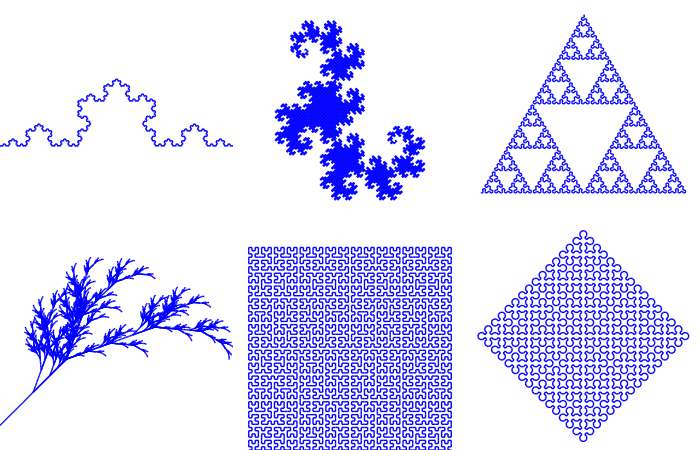# 分形与混沌

• 有着十分精细的不规则的结构
• 整体与局部相似，例如一根树杈的形状和一棵树很像

## Mandelbrot集合

Mandelbrot(曼德布洛特)集合是在复平面上组成分形的点的集合。

Mandelbrot集合的定义(摘自维基百科)

Mandelbrot集合可以用下面的复二次多项式定义：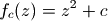Mandelbrot集合就是使以上序列不发散的所有c点的集合。

• 判断每次调用函数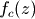得到的结果是否在半径R之内，即复数的模小于R
• 记录下模大于R时的迭代次数
• 迭代最多进行N次
• 不同的迭代次数的点使用不同的颜色绘制

```# -*- coding: utf-8 -*-

import numpy as np
import pylab as pl
import time
from matplotlib import cm

def iter_point(c):
z = c
for i in xrange(1, 100): # 最多迭代100次
if abs(z)>2: break # 半径大于2则认为逃逸
z = z*z+c
return i # 返回迭代次数

def draw_mandelbrot(cx, cy, d):
"""
绘制点(cx, cy)附近正负d的范围的Mandelbrot
"""
x0, x1, y0, y1 = cx-d, cx+d, cy-d, cy+d
y, x = np.ogrid[y0:y1:200j, x0:x1:200j]
c = x + y*1j
start = time.clock()
mandelbrot = np.frompyfunc(iter_point,1,1)(c).astype(np.float)
print "time=",time.clock() - start
pl.imshow(mandelbrot, cmap=cm.Blues_r, extent=[x0,x1,y0,y1])
pl.gca().set_axis_off()

x,y = 0.27322626, 0.595153338

pl.subplot(231)
draw_mandelbrot(-0.5,0,1.5)
for i in range(2,7):
pl.subplot(230+i)
draw_mandelbrot(x, y, 0.2**(i-1))
pl.subplots_adjust(0.02, 0, 0.98, 1, 0.02, 0)
pl.show()
```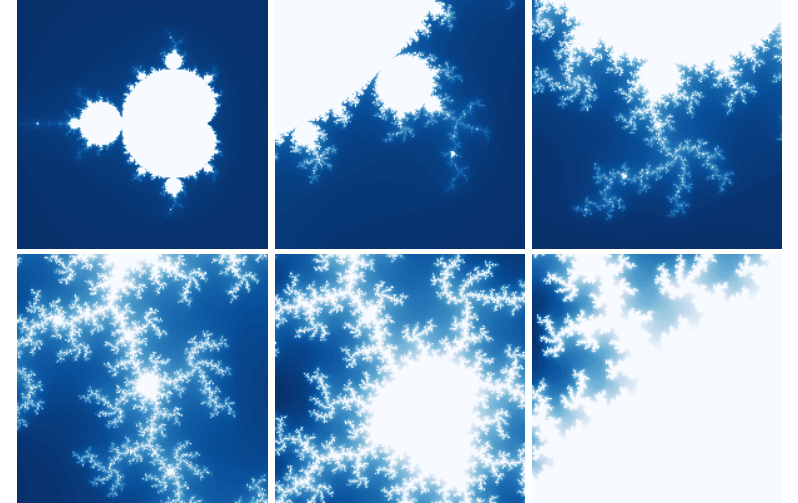Mandelbrot集合，以5倍的倍率放大点(0.273, 0.595)附近

```x0, x1, y0, y1 = cx-d, cx+d, cy-d, cy+d
y, x = np.ogrid[y0:y1:200j, x0:x1:200j]
c = x + y*1j
```

```mandelbrot = np.frompyfunc(iter_point,1,1)(c).astype(np.float)
```

```pl.imshow(mandelbrot, cmap=cm.Blues_r, extent=[x0,x1,y0,y1])
```

```time= 0.88162629608
time= 1.53712748408
time= 1.71502160191
time= 1.8691174437
time= 3.03812691278
```

```import scipy.weave as weave

def weave_iter_point(c):
code = """
std::complex<double> z;
int i;
z = c;
for(i=1;i<100;i++)
{
if(std::abs(z) > 2) break;
z = z*z+c;
}
return_val=i;
"""

f = weave.inline(code, ["c"], compiler="gcc")
return f
```

```time= 0.285266982256
time= 0.271430028118
time= 0.293769180161
time= 0.308515188383
time= 0.411168179196
```

```# -*- coding: utf-8 -*-

import numpy as np
import pylab as pl
import time
from matplotlib import cm

def draw_mandelbrot(cx, cy, d, N=200):
"""
绘制点(cx, cy)附近正负d的范围的Mandelbrot
"""
global mandelbrot

x0, x1, y0, y1 = cx-d, cx+d, cy-d, cy+d
y, x = np.ogrid[y0:y1:N*1j, x0:x1:N*1j]
c = x + y*1j

# 创建X,Y轴的坐标数组
ix, iy = np.mgrid[0:N,0:N]

# 创建保存mandelbrot图的二维数组，缺省值为最大迭代次数
mandelbrot = np.ones(c.shape, dtype=np.int)*100

# 将数组都变成一维的
ix.shape = -1
iy.shape = -1
c.shape = -1
z = c.copy() # 从c开始迭代，因此开始的迭代次数为1

start = time.clock()

for i in xrange(1,100):
# 进行一次迭代
z *= z
z += c
# 找到所有结果逃逸了的点
tmp = np.abs(z) > 2.0
# 将这些逃逸点的迭代次数赋值给mandelbrot图
mandelbrot[ix[tmp], iy[tmp]] = i

# 找到所有没有逃逸的点
np.logical_not(tmp, tmp)
# 更新ix, iy, c, z只包含没有逃逸的点
ix,iy,c,z = ix[tmp], iy[tmp], c[tmp],z[tmp]
if len(z) == 0: break

print "time=",time.clock() - start
pl.imshow(mandelbrot, cmap=cm.Blues_r, extent=[x0,x1,y0,y1])
pl.gca().set_axis_off()

x,y = 0.27322626, 0.595153338

pl.subplot(231)
draw_mandelbrot(-0.5,0,1.5)
for i in range(2,7):
pl.subplot(230+i)
draw_mandelbrot(x, y, 0.2**(i-1))
pl.subplots_adjust(0.02, 0, 0.98, 1, 0.02, 0)
pl.show()
```

```# 进行一次迭代
z *= z
z += c
```

```# 找到所有结果逃逸了的点
tmp = np.abs(z) > 2.0
# 将这些逃逸点的迭代次数赋值给mandelbrot图
mandelbrot[ix[tmp], iy[tmp]] = i
```

```# 找到所有没有逃逸的点
np.logical_not(tmp, tmp)
# 更新ix, iy, c, z只包含没有逃逸的点
ix,iy,c,z = ix[tmp], iy[tmp], c[tmp], z[tmp]
```

```time= 0.186070576008
time= 0.327006365334
time= 0.372756034636
time= 0.410074464771
time= 0.681048289658
time= 0.878626752841
```

### 连续的逃逸时间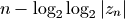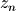是迭代n次之后的结果，通过在逃逸时间的计算中引入迭代结果的模值，结果将不再是整数，而是平滑渐变的。

```def smooth_iter_point(c):
z = c
for i in xrange(1, iter_num):
z = z*z+c
absz = abs(z)
if absz > 2.0:
mu = i - log(log(abs(z),2),2)
else:
mu = i
return mu # 返回正规化的迭代次数
```

```z = z*z+c
z = z*z+c
i += 2
```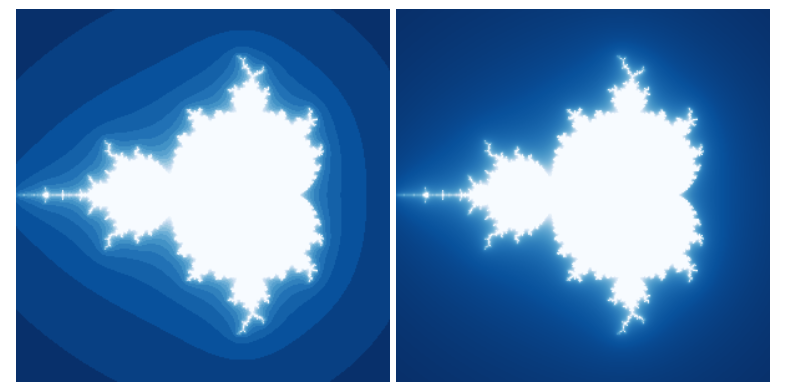## 迭代函数系统(IFS)

```1.
x(n+1）= 0
y(n+1) = 0.16 * y(n)

2.
x(n+1) = 0.2 * x(n) − 0.26 * y(n)
y(n+1) = 0.23 * x(n) + 0.22 * y(n) + 1.6

3.
x(n+1) = −0.15 * x(n) + 0.28 * y(n)
y(n+1) = 0.26 * x(n) + 0.24 * y(n) + 0.44

4.
x(n+1) = 0.85 * x(n) + 0.04 * y(n)
y(n+1) = −0.04 * x(n) + 0.85 * y(n) + 1.6
```

```# -*- coding: utf-8 -*-
import numpy as np
import matplotlib.pyplot as pl
import time

# 蕨类植物叶子的迭代函数和其概率值
eq1 = np.array([[0,0,0],[0,0.16,0]])
p1 = 0.01

eq2 = np.array([[0.2,-0.26,0],[0.23,0.22,1.6]])
p2 = 0.07

eq3 = np.array([[-0.15, 0.28, 0],[0.26,0.24,0.44]])
p3 = 0.07

eq4 = np.array([[0.85, 0.04, 0],[-0.04, 0.85, 1.6]])
p4 = 0.85

def ifs(p, eq, init, n):
"""
进行函数迭代
p: 每个函数的选择概率列表
eq: 迭代函数列表
init: 迭代初始点
n: 迭代次数

返回值： 每次迭代所得的X坐标数组， Y坐标数组， 计算所用的函数下标
"""

# 迭代向量的初始化
pos = np.ones(3, dtype=np.float)
pos[:2] = init

# 通过函数概率，计算函数的选择序列
rands = np.random.rand(n)
select = np.ones(n, dtype=np.int)*(n-1)
for i, x in enumerate(p[::-1]):
select[rands<x] = len(p)-i-1

# 结果的初始化
result = np.zeros((n,2), dtype=np.float)
c = np.zeros(n, dtype=np.float)

for i in xrange(n):
eqidx = select[i] # 所选的函数下标
tmp = np.dot(eq[eqidx], pos) # 进行迭代
pos[:2] = tmp # 更新迭代向量

# 保存结果
result[i] = tmp
c[i] = eqidx

return result[:,0], result[:, 1], c

start = time.clock()
x, y, c = ifs([p1,p2,p3,p4],[eq1,eq2,eq3,eq4], [0,0], 100000)
print time.clock() - start
pl.figure(figsize=(6,6))
pl.subplot(121)
pl.scatter(x, y, s=1, c="g", marker="s", linewidths=0)
pl.axis("equal")
pl.axis("off")
pl.subplot(122)
pl.scatter(x, y, s=1,c = c, marker="s", linewidths=0)
pl.axis("equal")
pl.axis("off")
pl.gcf().patch.set_facecolor("white")
pl.show()
```

```pos = np.ones(3, dtype=np.float)
pos[:2] = init
```

```p = np.add.accumulate(p)
rands = np.random.rand(n)
select = np.ones(n, dtype=np.int)*(n-1)
for i, x in enumerate(p[::-1]):
select[rands<x] = len(p)-i-1
```

```pl.scatter(x, y, s=1, c="g", marker="s", linewidths=0)
```

• s : 散列点的大小，因为我们要绘制10万点，因此大小选择为1
• c : 点的颜色，这里选择绿色
• marker : 点的形状，"s"表示正方形，方形的绘制是最快的
• linewidths : 点的边框宽度，0表示没有边框

```pl.scatter(x, y, s=1,c = c, marker="s", linewidths=0)
```### 2D仿射变换

```x(n+1) = A * x(n) + B * y(n) + C
y(n+1) = D * x(n) + E * y(n) + F
```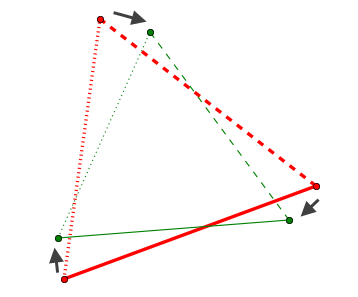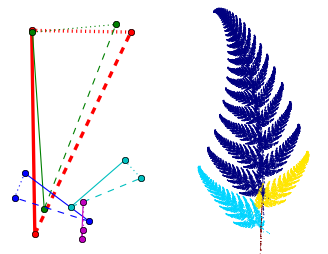5个三角形的仿射方程绘制蕨类植物的叶子

### 迭代函数系统设计器```def solve_eq(triangle1, triangle2):
"""
解方程，从triangle1变换到triangle2的变换系数
triangle1,2是二维数组：
x0,y0
x1,y1
x2,y2
"""
x0,y0 = triangle1
x1,y1 = triangle1
x2,y2 = triangle1

a = np.zeros((6,6), dtype=np.float)
b = triangle2.reshape(-1)
a[0, 0:3] = x0,y0,1
a[1, 3:6] = x0,y0,1
a[2, 0:3] = x1,y1,1
a[3, 3:6] = x1,y1,1
a[4, 0:3] = x2,y2,1
a[5, 3:6] = x2,y2,1

c = np.linalg.solve(a, b)
c.shape = (2,3)
return c
```

triangle_area函数计算三角形的面积，它使用NumPy的cross函数计算三角形的两个边的向量的叉积：

```def triangle_area(triangle):
"""
计算三角形的面积
"""
A = triangle
B = triangle
C = triangle
AB = A-B
AC = A-C
return np.abs(np.cross(AB,AC))/2.0
```

IFSDesigner._figure_default创建Figure对象，并且添加两个并排的子图ax和ax2，ax用于三角形编辑，而ax2用于分形图案显示。

```def _figure_default(self):
"""
figure属性的缺省值，直接创建一个Figure对象
"""
figure = Figure()
self.ax2.set_axis_off()
self.ax.set_axis_off()
figure.patch.set_facecolor("w")
return figure
```

IFSTriangles类完成三角形的编辑工作，其中通过如下的语句绑定Figure控件的canvas的鼠标事件

```canvas = ax.figure.canvas
# 绑定canvas的鼠标事件
canvas.mpl_connect('button_press_event', self.button_press_callback)
canvas.mpl_connect('button_release_event', self.button_release_callback)
canvas.mpl_connect('motion_notify_event', self.motion_notify_callback)
```

```class IFSHandler(Handler):
"""
在界面显示之前需要初始化的内容
"""
def init(self, info):
info.object.init_gui_component()
return True
```

```def init_gui_component(self):
self.ifs_triangle = IFSTriangles(self.ax)
self.figure.canvas.draw()
...
```

```def ifs_calculate(self):
"""
在别的线程中计算
"""
def draw_points(x, y, c):
if len(self.ax2.collections) < ITER_TIMES:
try:
self.ax2.scatter(x, y, s=1, c=c, marker="s", linewidths=0)
self.ax2.set_axis_off()
self.ax2.axis("equal")
self.figure.canvas.draw()
except:
pass

def clear_points():
self.ax2.clear()

while 1:
try:
if self.exit == True:
break
if self.clear == True:
self.clear = False
self.initpos = [0, 0]
x, y, c = ifs( self.ifs_triangle.get_areas(),
self.ifs_triangle.get_eqs(), self.initpos, 100)
self.initpos = [x[-1], y[-1]]
self.ax2.clear()

x, y, c = ifs( self.ifs_triangle.get_areas(),
self.ifs_triangle.get_eqs(), self.initpos, ITER_COUNT)
if np.max(np.abs(x)) < 1000000 and np.max(np.abs(y)) < 1000000:
self.initpos = [x[-1], y[-1]]
wx.CallAfter( draw_points, x, y, c )
time.sleep(0.05)
except:
pass
```

```self.version += 1
```

```@on_trait_change("ifs_triangle.version")
def on_ifs_version_changed(self):
"""
当三角形更新时，重新绘制所有的迭代点
"""
self.clear = True
```

## L-System分形

• F : 向前走固定单位
• + : 正方向旋转固定单位
• - : 负方向旋转固定单位

```F+F--F+F
```

```F+F--F+F+F+F--F+F--F+F--F+F+F+F--F+F
```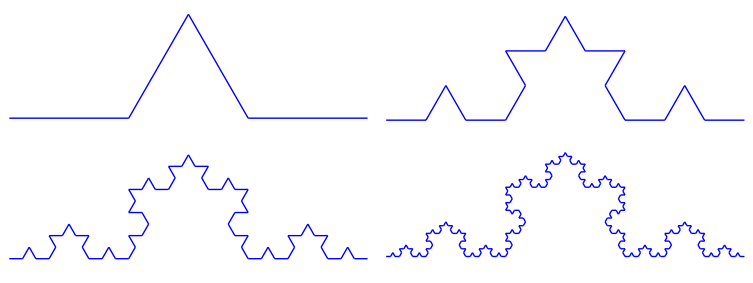• f : 向前走固定单位，为了定义不同的迭代公式
• [ : 将当前的位置入堆栈
• ] : 从堆栈中读取坐标，修改当前位置
• S : 初始迭代符号

```S -> X
X -> F-[[X]+X]+F[+FX]-X
F -> FF
```

```{
"X":"F-[[X]+X]+F[+FX]-X", "F":"FF", "S":"X",
"direct":-45,
"angle":25,
"iter":6,
"title":"Plant"
}
```

• direct : 是绘图的初始角度，通过指定不同的值可以旋转整个图案
• angle : 定义符号+,-旋转时的角度，不同的值能产生完全不同的图案
• iter : 迭代次数

```class L_System(object):
def __init__(self, rule):
info = rule['S']
for i in range(rule['iter']):
ninfo = []
for c in info:
if c in rule:
ninfo.append(rule[c])
else:
ninfo.append(c)
info = "".join(ninfo)
self.rule = rule
self.info = info

def get_lines(self):
d = self.rule['direct']
a = self.rule['angle']
p = (0.0, 0.0)
l = 1.0
lines = []
stack = []
for c in self.info:
if c in "Ff":
r = d * pi / 180
t = p + l*cos(r), p + l*sin(r)
lines.append(((p, p), (t, t)))
p = t
elif c == "+":
d += a
elif c == "-":
d -= a
elif c == "[":
stack.append((p,d))
elif c == "]":
p, d = stack[-1]
del stack[-1]
return lines
```

```import matplotlib.pyplot as pl
from matplotlib import collections

# rule = {...}  此处省略rule的定义

lines = L_System(rule).get_lines()

fig = pl.figure()